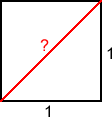# The Dangerous Ratio

##### Age 11 to 14

Published 2004 Revised 2009It's a stormy day on the sea off the coast of Greece. The date is around 520 BC. Fighting for his life, a man is heaved over the side of a boat and dropped into the open water to die. His name is Hippasus of Metapontum. His crime? Telling the world a mathematical secret. The secret of the dangerous ratio.

The murder of Hippasus is a matter of legend, but the secret was real, and certainly dangerous enough to the beliefs of those who knew about it.

It was a secret owned by the school of Pythagoras. These early Greek mathematicians (Pythagoras himself was born around 569 BC ) were obsessed with the significance of whole numbers and their ratios. The Pythagorean's motto, carved above the entrance of the school, was "All is number".

The inner circle of the school, the mathematikoi, believed that the universe was built around the whole numbers. Each number from one to ten was given a very special significance. Odd numbers were thought to be male and even numbers female. Yet there was one number that the Pythagoreans found terrifying, the number that might have cost Hippasus his life for revealing its existence to the world.

The name Pythagoras these days is best remembered for a geometrical theorem, the one that tells us how to calculate the lengths of the sides of a right angled triangle, and it is from this theorem that the dangerous ratio emerges.

Imagine a simple square shape, each side 1 unit in length. How long is the square's diagonal?This seemingly harmless question was the trigger for the Pythagoreans' disturbing discovery. The length of the square's diagonal is easy to work out. It forms the long side of a triangle with a right angle opposite, and two other sides of length 1 unit. Thanks to Pythagoras' theorem we (and the Greeks) know that we can work out the square of the length of the longest side of a right-angled triangle by adding together the squares of the other two sides. So we know the diagonal's length squared is $(1 \times 1) + (1 \times 1) = 2$, making the length of the diagonal itself $\surd2$. The number which when multiplied by itself makes 2. But what is that number?

The square root of 2 isn't 1 because 1x1 is 1.

And it isn't 2, because 2x2 is 4.

It's something in between.

This wasn't a problem for the Pythagoreans. It was obviously a ratio of two whole numbers. They only had to figure out what that ratio was. At least that was the theory.

But after more and more frantic attempts, a horrible discovery was made. There is NO ratio that will produce $\surd 2$ - it simply can't be done. It's what we now call an irrational number, not because it is illogical, but because it can't be represented as a ratio of whole numbers.

This was what sent the Pythagoreans into such a spin that they may have sacrificed poor Hippasus. If you believe that everything is constructed from whole numbers, it is a terrible a shock to discover that there is an everyday number, a 'real world' number like the diagonal of a square, that doesn't fit your picture of the world. It's a nightmare - and one from which the Pythagoreans would never really recover.

How is it possible to prove that there is no ratio making $\surd 2$?

The logic is a little fiddly, but not too heavy.

Let's imagine that it is possible to come up with such a ratio to produce $\surd 2$.

Let's call it $\frac {top}{bottom}$.

Make this the simplest ratio you can have - cancelling out any common factors.

Now, let's multiply both sides of the equation by itself, so

$\frac {top^2}{bottom^2} = 2$

Next multiply both sides by $bottom^2$, ending up with

$top^2 = 2 \times bottom^2$

This means that $top^2$ must be an even number - because 2 times anything is even.

And that makes top an even number too - because an odd number multiplied by itself is always odd.

What's more, if $top^2$ is an even number, so is $bottom^2$.

This is because an even number squared divided by 2 is still even - and $bottom^2$ is $top^2$ divided by $2$.

That makes bottom an even number. So, top and bottom are both even.

That means that each of them can be divided by 2.

But hang on. We started out by saying top and bottom were chosen as the simplest possible ratio - any common factors had already been divided out.

Now we are saying they have to have a common factor of 2. This isn't possible. The only thing we can have wrong is our original assumption, which was that $\surd 2$ is a rational number. You simply can't have a ratio that makes up $\surd 2$. As Hippasus discovered to his cost, that inscription over the Pythagorean school All is number would have to be extended to cope with more complex ideas than ratios of whole numbers.

[Editor's note: If you have enjoyed this article you might like to try this interactivity . It is based on a slightly different proof that the square root of 2 is irrational. This alternative proof can be generalised to prove that all square roots of whole numbers that are not square numbers are irrational, that is the square roots of 3, 5, 6, 7, 8, 10, 11 ...etc.]